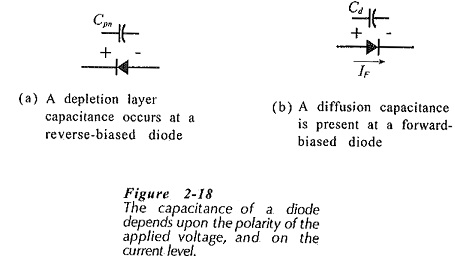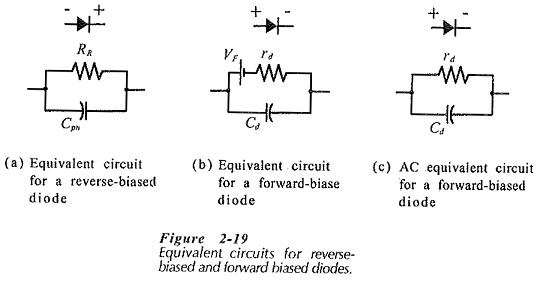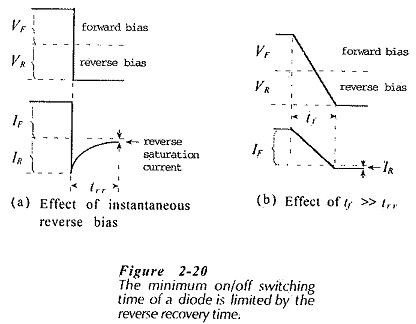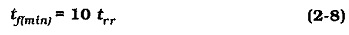# AC Equivalent Circuit of Semiconductor Diode

### AC Equivalent Circuit of Semiconductor Diode:

Junction Capacitances – The depletion region of a pn-junction is a layer depleted of charge carriers situated between two blocks of low resistance material. Because this is the description of a capacitor, the depletion region clearly has a capacitance. The depletion layer capacitance (Cpn) may be calculated from the equation for a parallel-plate capacitor if the junction dimensions are known. Typically, Cpn is 4 pF for a low-current diode.The depletion layer capacitance is essentially the capacitance of a reverse-biased pn-junction, [Fig. 2-18(a)]. Consider the forward-biased junction in Fig. 2-18(b). If the applied voltage is suddenly reversed, forward current IF ceases immediately leaving some majority charge carriers in the depletion region. These charge carriers must flow back out of the depletion region, which is widened when the junction is reverse biased. The result is that, when a forward-biased junction is suddenly reversed, a reverse current flows which is large initially and slowly decreases to the level of the reverse saturation current. The effect may be likened to the discharging of a capacitor, and so it is represented by a capacitance known as the diffusion capacitance (Cd).

It can be shown that Cd is proportional to the forward current IF. This is to be expected, since the number of charge carriers in the depletion region must be directly proportional to IF . For a low-current diode with a 10 mA forward current, a typical value of diffusion capacitance is around 1 nF.

### AC Equivalent Circuits (Reverse- and Forward-Biased):

A reverse-biased diode can be simply represented by the (high non-constant) reverse resistance RR in parallel with the depletion layer capacitance Cpn, [Fig. 2-19(a)]. The AC Equivalent Circuit of Semiconductor Diode for a forward-biased diode consists of the dynamic resistance rd in series with a voltage cell representing VF. To allow for the effect of the diffusion capacitance, Cd is included in parallel. This gives the complete equivalent circuit shown in Fig. 2-19(b).The equivalent circuit for the forward-biased diode may be modified to form an AC Equivalent Circuit of Semiconductor Diode. This applies to diodes that are maintained in a forward-biased condition while subjected to small variations in IF and VF. The AC Equivalent Circuit of Semiconductor Diode is created simply by removing the voltage cell representing VF from the complete equivalent circuit, [see Fig. 2-19(c)].

### Reverse Recovery Time:

In many applications, diodes must switch rapidly between forward and reverse bias. Most diodes switch very quickly into the forward-biased condition, however, there is a longer turn-off time due to the junction diffusion capacitance.Figure 2-20 illustrates the effect of a voltage pulse on the diode forward current. When the pulse switches from positive to negative, the diode conducts in reverse instead of switching off sharply, [Fig. 2-20(a)). The reverse current (IR) initially, equals the forward current (IF), then it gradually decreases toward zero. The high level of reverse current occurs because at the instant of reverse bias there are charge carriers crossing the junction depletion region, and these must be removed. (The same effect that produces diffusion capacitance.) The reverse recovery time (trr) is the time required for the current to decrease to the reverse saturation current level. Typical values of trr for switching diodes range from 4 ns to 50 ns. Figure 2-20(b) shows that, to keep the diode reverse current to a minimum, the fall time (tf) of the applied voltage pulse must be much larger than the diode trr. Typically,Updated: January 28, 2019 — 10:25 pm